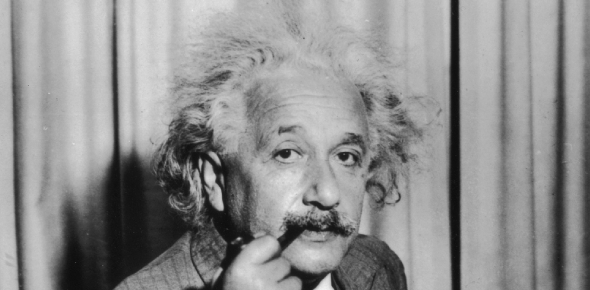How does Einstein's prove the Pythagorean Theorem? - ProProfs DiscussTopicsMoreProductsMore+ Ask Question

# How does Einstein's prove the Pythagorean Theorem?

Asked by K. Tanaka, Last updated: Mar 29, 2020

###Request 0FollowShareAnswer AnonymouslyAnswer LaterCopy Link#### John F. connor

Traveler, Avid Reader, Free as a bird

John F. connor, Content Marketing executive, MA, Minsk,PolandAlbert Einstein was a well-known scientist and mathematician. Even though the Pythagorean theorem was founded long before Einstein was born, he still played a part in its proof. Einstein wrote a mathematical proof using the Pythagorean theorem. Some people consider it to be the most excellent proof. When presented with a regular triangle, Einstein drew a line straight down to create two right triangles from the one big triangle.All three triangles were considered similar. His proof was able to prove that the first big triangle is now the hypotenuse of one of the smaller triangles. Einstein is able to prove this by using all three triangles. Many people not only use the Pythagorean Theorem, but they also use Einstein’s proof of it to this day.Search for Google imagesSelect a recommended image
Upload from your computerCancelSearch for Google imagesSelect a recommended image
Upload from your computerCancelSearch for Google imagesSelect a recommended image
Upload from your computerCancel